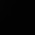## Tuesday, 5 June 2012

### NCERT CHAPTER SOLUTIONS

Exercise 1.1 (Rational Numbers)

Q1: Is zero a rational number? Can you write it in the form p/q, where p and q are integers and q ≠ 0?

Answer: Yes zero is a rational number. It can be represented as p/q form e.g. 0/1, 0/2, 0/15 etc.

Q2: Find six rational numbers between 3 and 4.

Answer:  Between 3 and 4, one can have infinite rational numbers.
E.g. 3 and 4 can be represented as p/q form as 33/11 and 44/11.

We can have, 34/11, 35/11, 36/11, 37/11, 38/11 and 39/11Q4: State whether the following statements are true or false. Give reasons for your answers.

(i) Every natural number is a whole number. (TRUE)
Explanation: Whole numbers contains all natural numbers.

(ii) Every integer is a whole number. (FALSE)
Explanation: Since integers include negative numbers while whole numbers do not.

(iii) Every rational number is a whole number. (FALSE)
Explanation: E.g. 3/5 a rational number cannot be represented either as whole number.

Few properties of Rational Numbers
1. If x, y are rational numbers, then x + y = y + x. (Commutative Property of addition)
2. If x, y, z are rational numbers, then x + (y + z) = (x + y) + z. (associative property of addition).
3. The rational number 0 is called the additive identity of rational number..
4. For every rational number x, there is a rational number −x such that x + (−x) = (−x) + x = 0. The rational number –x is called  additive inverse of rational number.
5. If x, y are rational numbers, then xy = yx. (Commutative Property of multiplication)
6. If x, y, z are rational numbers, then x(yz) = (xy)z. (associative property of multiplication)
7. For every non-zero rational number x, 1/x is a rational number which is its multiplicative inverse.
Q5(CBSE): Define Rational Number.

Answer:  A rational number is a number which can be expressed in the form of p/q, where p and q are integers without and common factor between them. And q is not zero.
Q = {x|x = p/q, p,q ∈ I and q ≠ 0}

A rational number may be positive, negative or zero.

Q6: What is the smallest natural number?

Q7: What is the smallest whole number?

Q8: What is the additive inverse of -4/5?

Q9: What is the multiplicative inverse of -3/8?

Q10: List an integer which is not a natural number?

Answer: 0, all -ve numbers are integers but not natural numbers.

Q11: Is zero (0) a rational number?

Answer: Yes. Since we can write 0 in p/q form i.e. 0/11.The word "associative" comes from "associate" or "group";the Associative Property of Addition is the rule that refers to grouping. For addition, the rule is "a + (b + c) = (a + b) + c"; in numbers, this means
2 + (3 + 4) = (2 + 3) + 4.

2.sooooooooooooooooooooooooooooooooooooooooooooooooooooooooooooooooooooooooooooooooooooo gggggggggggggggggggoooooooooooooooooooooooddddddddddddddddddddddddddddddddddddddddddddddddddddddddd sssssssssssssssssssssssssssssssssiiiiiiiiiiiiiiiiiiiiiiiiiiiiiiiiiiiiiiiittttttttttttttttttttttttttttttttttttttttttttteeeeeeeeeeeeeeeeeeeeeeee

3.4.